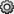## Display Statistics in the Workspace Browser

For each variable or object, the Workspace browser can display statistics such as the Min, Max, and Mean, when relevant. MATLAB® performs these calculations using the `min`, `max`, and `mean` functions, and updates the results automatically.

To display statistics, on the Workspace browser title bar, click the Show Workspace Actionsbutton, and then select Choose Columns. Select the statistics you want MATLAB to calculate. In MATLAB Online™, to select which columns to display, right-click a column name in the Workspace panel and select or clear the desired column names.

### Improve Workspace Browser Performance During Statistical Calculations

If you show statistical columns in the Workspace browser, and you work with very large arrays, you might experience performance issues when the data changes as MATLAB updates the statistical results. To improve performance, consider one or both of the following:

• Show only the statistics of interest to you.

On the Workspace browser title bar, click the Show Workspace Actionsbutton, and then select Choose Columns. Clear the statistics that you do not want MATLAB to calculate. In MATLAB Online, to change which statistics display, right-click a column name in the Workspace panel and clear the statistics that you do not want MATLAB to calculate.

• Exclude large arrays from statistical calculations.

On the Home tab, in the Environment section, click the Specify preferencesbutton. Select MATLAB > Workspace, and then use the arrow buttons under Statistical calculations to change the maximum array size for which the Workspace browser performs statistical calculations. Any variable exceeding the maximum array size reports `<Too many elements>` in Workspace browser statistics columns instead of statistical results.

### Include or Exclude NaN Values in Statistical Calculations

If your data includes `NaN`s (Not-a-Number values), you can specify that the Workspace browser statistical calculations consider or ignore the `NaN`s. On the Home tab, in the Environment section, click the Specify preferencesbutton. Select MATLAB > Workspace, and then select one of the following:

• Use NaNs when calculating statistics

If a variable includes a `NaN`, and you select this option, the values for Min, Max, Var, and several other statistics appear as `NaN`. However, Mode and several other statistics show a numeric result.

• Ignore NaNs whenever possible

If a variable includes a `NaN`, and you select this option, numeric results appear for most statistics including Min, Max, and Mode. However, Var still appears as `NaN`.